# Question:How to compute the restricted-edge-connectivity of a given graph (there is a polynomial algorithm)?

## Question:How to compute the restricted-edge-connectivity of a given graph (there is a polynomial algorithm)?

Maple 2021
！Edits: I have found  a existing polynomial algorithm, but I still have difficulty implementing it. 2022/10/26

The edge connectivity of a connected graph G  is the minimum number of edges whose deletion from the graph G disconnects G. Below we are concerned with a particular kind of edge-cut.

• For a connected graph G=(V ,E), an edge set S ⊂ E is a restricted-edge-cut, if G−S is disconnected and every connected component of  G−S has at least 2 vertices.

Clearly, a restricted-edge-cut is an edge cut with a special requirement.

• The restricted-edge-connectivity of G, denoted by κres(G), is defined as the cardinality of a minimum restricted-edge-cut.

For example, a graph g is as follows.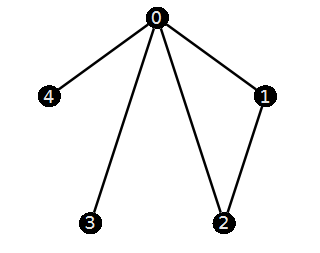Clearly, its edge-connectivity is 1 since (0,3) or (0,4) is a edge cut of g. But we can find that  if we remove the edge (0,3), then "3" is a isolated vertex. Similarly, "4" is a isolated vertex if we remove  (0,4). It is not difficult to find g has exactly the two cut-edges (0,3) and (0,4).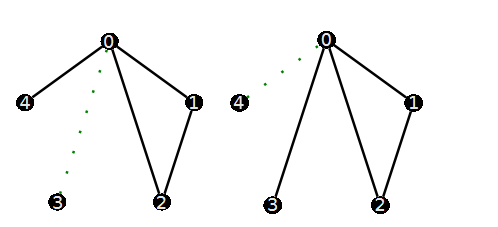Based on the definition of the restricted-edge-cuts, neither {(0,3)} nor  {(0,4)} are restricted-edge-cuts A minimum restricted-edge-cut is {(0,1),(0,2)} since every connected component of G-{(0,1),(0,2)} has  (at least) 2 vertices.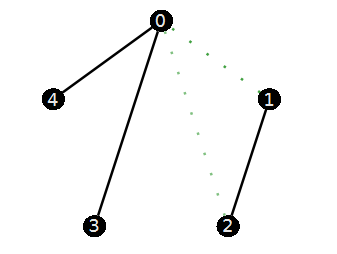So  κres(g) is 2.  My problem is:

Given a graph G, how to calculate the restricted-edge-connectivity of  G?  Moreover, how to find a minimum restricted-edge-cut?

A specific graph that I want to test is as follows. (it has 16 vertices and 56 edges.) I would like to calculate its restricted-edge-connectivity and find a minimum restricted-edge-cut.

```g:=ConvertGraph("O~tIID@wL~j`PbOqgLJ@p");
vertexcolor="Black",edgethickness=2])
```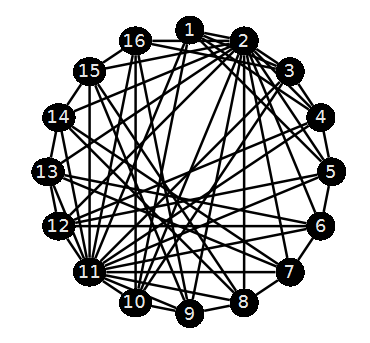`EdgeConnectivity(g) `

6

One option I came up with is to find all 6-edge subsets first. Test if they satisfy  the restricted condition (one by one). Then continue to increase to 7 or more. But this violent calculation may get stuck in the first step. That is, test all the minimum edge cut sets (Note that we will consider 32468436 edge-subsets!) I was referring to the efficiency aspect.

```with(Iterator):
C:=Combination(58,6):
K:=Edges(g):
#sub:=seq( K[[seq(c[]+1)]], c=C); # do not run this code since it has 32468436 members.```

Any language is acceptable.( C or C++, Python. )

PS: Some time ago, I also asked a related question (but with some differences) on mathematica stack （Find all the minimum edge cuts of a graph）. Although Bob Hanlon  gave a reply, the actual result is not good.

Edits: The following literature gives a polynomial algorithm for computing the restricted-edge-connectivity of a given  graph. The heart of it is to computing the least cardinality of some  edge-pairs's edge separator. I'm stuck here.

• Esfahanian A H, Hakimi S L. On computing a conditional edge-connectivity of a graph[J]. Information processing letters, 1988, 27(4): 195-199.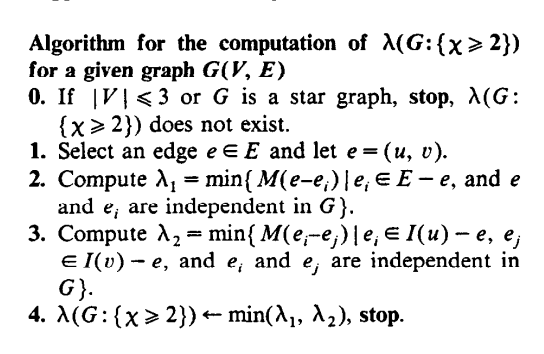How to implement this algorithm is my current concern.﻿